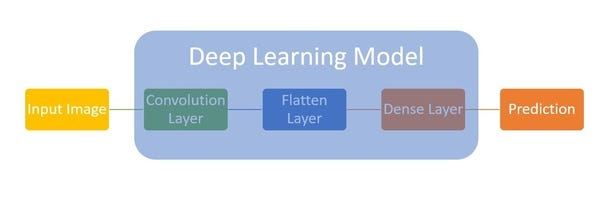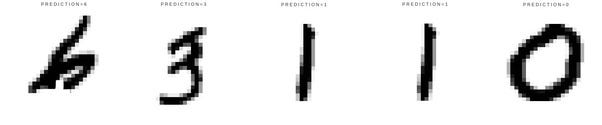# “Hello World!” of Deep Learning

Source: Deep Learning on MediumHow to Write “Hello World!” of Deep Learning

This article presents the first program for deep learning enthusiasts. We’ll use Google’s free cloud service to avoid the hassles of installing softwares and packages. A simple program will help the user with minimum code and a meaningful application in 3 simple steps.

### 1. Define Model

For a deep learning model, all we need to decide is number of layers and number of neurons in each layer. MNIST images have 28×28 pixels, those become neurons for the first layer. Let’s add convolutional layer, followed by flatten layer and dense layers to create our deep learning model. With that our network looks somewhat like shown belowDeep Learning Model for MNIST “Hello World!”

We use python and Kerars (a high-level neural networks API) to implement the model we designed above. Code for such a network is given below:

`model = Sequential();model.add(Conv2D(32, kernel_size=(3, 3), activation='relu', \          input_shape=input_shape)) model.add(Flatten());model.add(Dense(num_classes, activation='softmax'))`

### 2. Train Model

Now, it’s time to train our model. Training the model is as simple as a single function call

`model.fit(x_train, y_train, batch_size=batch_size, epochs=2, \          validation_data=(x_test, y_test))`

### 3. Display “Hello !”

Now that we are done with training, Pyplot package helps us display the digit image prediction along with images. So here is DL model saying “h e l l o”, with MNIST digit recognition.MNIST CNN Says “Hello”

Here is the link to python source code to run on google GPUs. For those unfamiliar with googles free cloud service, here is a friendly tutorial on google colab.

Have fun playing with the code. 👩‍💻

References: